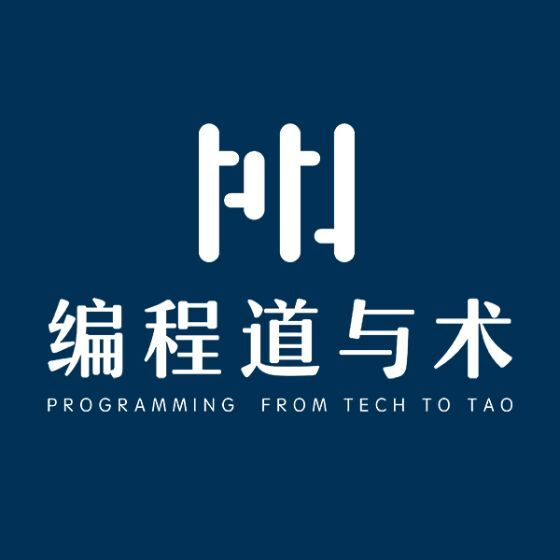# 原创 | 正确区分属性和字段﻿﻿## 范例

package yang.yu.training;import javax.persistence.Embeddable;import java.io.Serializable;import java.util.Objects;@Embeddablepublic class Point implements Serializable {    private int x;    private int y;    public Point(int x, int y) {        this.x = x;        this.y = y;    }    public int getX() {        return x;    }    public void setX(int x) {        this.x = x;    }    public int getY() {        return y;    }    public void setY(int y) {        this.y = y;    }    @Override    public boolean equals(Object other) {        if (this == other) {            return true;        }        if (!(other instanceof Point)) {            return false;        }        Point that = (Point) other;        return this.x == that.x &&                this.y == that.y;    }    @Override    public int hashCode() {        return Objects.hash(x, y);    }    @Override    public String toString() {        return "Point(" + x + ", " + y + ")";    }    public static Point of(int x, int y) {        return new Point(x, y);    }}

package yang.yu.training;import javax.persistence.Entity;import javax.persistence.Id;import java.io.Serializable;import java.util.Objects;import java.util.UUID;@Entitypublic class Rectangle implements Serializable {    @Id    private String id = UUID.randomUUID().toString();    private Point lowerLeftCoordinate;    private int _width;    private int _height;    public Rectangle(int width, int height) {        this._width = width;        this._height = height;        lowerLeftCoordinate = new Point(0, 0);    }    public String getId() {        return id;    }    public int getWidth() {        return _width;    }    public int getHeight() {        return _height;    }    public int getArea() {        return _width * _height;    }    public boolean isSquare() {        return _width == _height;    }    public Point getLowerLeftCoordinate() {        return lowerLeftCoordinate;    }    public Point getLoweRightCoordinate() {        return new Point(lowerLeftCoordinate.getX() + _width, lowerLeftCoordinate.getY());    }    public Point getUpperLeftCoordinate() {        return new Point(lowerLeftCoordinate.getX(), lowerLeftCoordinate.getY() + _height);    }    public Point getUpperRightCoordinate() {        return new Point(lowerLeftCoordinate.getX() + _width, lowerLeftCoordinate.getY() + _height);    }    public void moveHorizontally(int delta) {        lowerLeftCoordinate = new Point(lowerLeftCoordinate.getX() + delta, lowerLeftCoordinate.getY());    }    public void moveVertically(int delta) {        lowerLeftCoordinate = new Point(lowerLeftCoordinate.getX(), lowerLeftCoordinate.getY() + delta);    }    public void moveTo(Point point) {        lowerLeftCoordinate = new Point(lowerLeftCoordinate.getX() + point.getX(), lowerLeftCoordinate.getY() + point.getY());    }    @Override    public boolean equals(Object other) {        if (this == other) {            return true;        }        if (!(other instanceof Rectangle)) {            return false;        }        Rectangle that = (Rectangle) other;        return this._width == that._width &&                this._height == that._height;    }    @Override    public int hashCode() {        return Objects.hash(_width, _height);    }    @Override    public String toString() {        return "Rectangle{" +                "_width=" + _width +                ", _height=" + _height +                '}';    }}

## 属性和字段的定义

private int x;    public int getX() {        return x;    }    public void setX(int x) {        this.x = x;    }private int y;    public int getY() {        return y;    }    public void setY(int y) {        this.y = y;    }

private int x;

public int getX() {        return x;    }    public void setX(int x) {        this.x = x;    }

## 属性和字段之间没有必然的一一对应关系

在一个类之中，属性和字段没有必然的一一对应关系。

### 属性和字段的名称可以是不同的

private int _width;         //定义字段_width    public int getWidth() {     //定义属性width        return _width;    }

### 有的字段没有对应的属性，有的属性没有对应的字段

public int getArea() {        return _width * _height;    }

private Point lowerLeftCoordinate;    public Point getLowerLeftCoordinate() {        return lowerLeftCoordinate;    }

public Point getLoweRightCoordinate() {        return new Point(lowerLeftCoordinate.getX() + _width, lowerLeftCoordinate.getY());    }    public Point getUpperLeftCoordinate() {        return new Point(lowerLeftCoordinate.getX(), lowerLeftCoordinate.getY() + _height);    }    public Point getUpperRightCoordinate() {        return new Point(lowerLeftCoordinate.getX() + _width, lowerLeftCoordinate.getY() + _height);    }

### 有的属性是多个字段综合表现的结果

public boolean isSquare() {        return _width == _height;    }

public int getArea() {        return _width * _height;    }

### 属性可以是只读、只写或读写的，而字段都是可读写的

private int _width;    private int _height;    public Rectangle(int width, int height) {        this._width = width;        this._height = height;        lowerLeftCoordinate = new Point(0, 0);    }    public int getWidth() {        return _width;    }    public int getHeight() {        return _height;    }

## 属性是类外部接口的一部分，字段是类内部实现的一部分

属性是类外部接口的一部分；字段是类内部实现的一部分。

属性是你的代码和你代码的客户的协议的一部分，必须精心设计，不可随意改动；而字段属于你的自由王国，你可以随意添加、删除和修改，只要不影响类的外部接口。

外部接口的“形诸外”是内部实现的“动于内”的结果。

## 重要推论

面向接口编程，不要面向实现编程。

### 先定义属性而不是字段

先定义属性（因为它是类接口的一部分），然后根据需要定义字段，而不是相反。

### 不要为每个字段默认生成属性

千万不要、不要、不要不假思索地为每个字段添加getter和setter方法生成属性。

### 尽量通过方法而不是setter来修改字段值

尽量通过真正的方法而不是通过setter修改字段值。

public void moveHorizontally(int delta) {        lowerLeftCoordinate = new Point(lowerLeftCoordinate.getX() + delta, lowerLeftCoordinate.getY());    }    public void moveVertically(int delta) {        lowerLeftCoordinate = new Point(lowerLeftCoordinate.getX(), lowerLeftCoordinate.getY() + delta);    }    public void moveTo(Point point) {        lowerLeftCoordinate = new Point(lowerLeftCoordinate.getX() + point.getX(), lowerLeftCoordinate.getY() + point.getY());    }

public void setLowerLeftCoordinate(Point newCoordinate) {        this.lowerLeftCoordinate = lowerLeftCoordinate;    }

1. 隐藏内部实现。不必将“通过修改左下角坐标来实现矩形的移动”这样的内部实现细节呈现给代码的用户。

2. 面向意图编程。因为用户的意图就是将矩形移动到一个新的位置，而不是重新设置它的左下角坐标。

## JPA/Hibernate中区分属性和字段持久化

JPA/Hibernate中，如果@Id注解如果定义在属性上，就是根据属性进行持久化的；如果是定义在字段上，就是根据字段来进行持久化的。

@Entitypublic class Rectangle {    @Id    private String id;    private int _width;    public String getId() {        return id;    }    public int getWidth() {        return _width;    }

SELECT o FROM Rectangle o WHERE o._width > 100

SELECT o._width, o._height WHERE o._width > 100

@Entitypublic class Rectangle {    private String id;    private int _width;    @Id    public String getId() {        return id;    }    public int getWidth() {        return _width;    }

SELECT o FROM Rectangle o WHERE o.width > 100

SELECT o.width, o.height WHERE o.width > 100

@Id字段定义在字段还是属性上决定了整个类的所有属性/字段的持久化策略。

## 深入思考

﻿

﻿## 评论